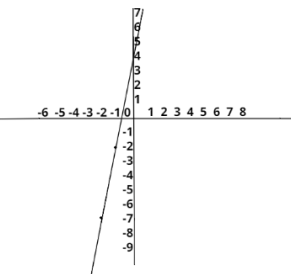Courses
Courses for Kids
Free study material
Free LIVE classes
MoreLIVE
Join Vedantu’s FREE Mastercalss

# The graph of p(x) = 5x + 3, where is?A. A RayB. A parabolaC. A line segmentD. A lineVerified
361.5k+ views
Hint: Draw a graph of a given function by assuming values of x and substituting in function to get values of y and using both x and y values plot the graph in x-y coordinate.

Given,
p(x)=5x+3
On observing carefully, we come to know that it’s the equation of the type:
y= mx + c

And we see it’s a linear equation in two variables we can denote those variables in x – axis and y - axis.

If we want to do cross check, we can simply put the real value of x we will always get the real value of y
The value of x and y will denote the coordinate on joining that coordinate we will be given a straight line.

Now let's check it on a graph when x = -1 then y = -2 and when x = -2 then y = -7 plot these points on the graph and check what it is.Yes, it is a straight line.

Note: Whenever you strike with this type of problem, always think about the degree of the variable. If it is 1 then it must be a straight line otherwise use various methods for checking the graph and get the results.
Last updated date: 24th Sep 2023
Total views: 361.5k
Views today: 4.61k